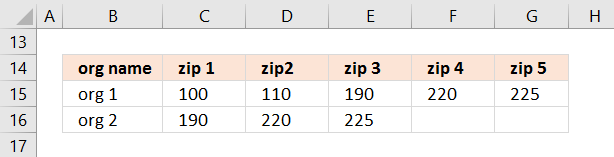Author: Oscar Cronquist Article last updated on September 07, 2021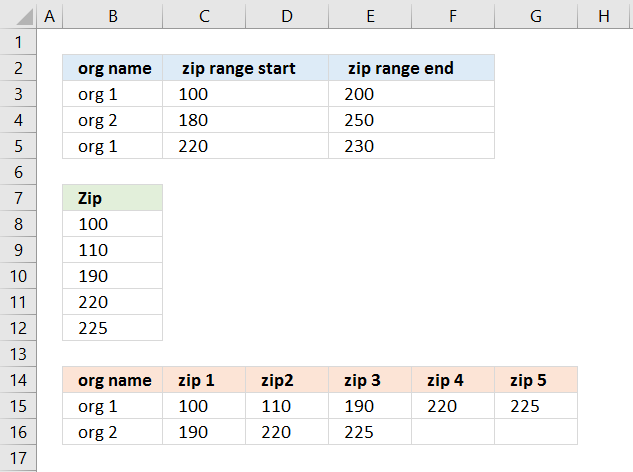This article demonstrates how to distribute values into specific ranges with possible overlapping ranges. I have written articles about filter rows based on a range criteria and extract records between two dates if you are interested in how to perform lookups based on ranges.

This example, demonstrated in the image above, shows ranges defined in cell B2:F5, note that range "org2" 180-250 partially overlaps "org1" 100-200 and 220-230.

Example values are in cell range B8:B12, they are 100, 110, 190, 220, and 225.

There are two formulas, the first one creates a unique distinct list in cell B15 and cells below. The second formula distributes values across ranges based on item names on the same row in column B, a value may exist in two or more ranges simultaneously.

Value 100 is only in item name "org 1", however, value 190 is in both "org 1" and "org 2". Value 190 matches both range 100-200 and 180-250.

### 1. Question

Thank you *so* much for your detailed examples and for actively replying to users! I have a problem, which I've tried solving by editing your formula examples for 20+ hours without success although I thought I could do it myself but apparently not, so here goes:I have a long list of organizations that work in specific zip areas defined by zip ranges (start and end). One org might have multiple zip ranges and there can be overlap between organizations (i.e. one zip might "belong" to >1 org). Then there's another list that has got all the possible existing zips. I would need to have all existing zips falling inside the zip range of the organization added to separate columns on the matching row of the first list.First list:
org name | zip range start | zip range end
org 1 | 00100 | 00200
org 2 | 00180 | 00250
org 1 | 00220 | 00230Second list:
00100
00110
00190
00220
00225Desired result:
org name | zip1 | zip2 | zip3 | zip n...
org 1 | 00100 | 00110 | 00190
org 2 | 00190 | 00220 | 00225
org 1 | 00220 | 00225Perfect result:
org name | zip1 | zip2 | zip3 | zip n...
org 1 | 00100 | 00110 | 00190 | 00220 | 00225
org 2 | 00190 | 00220 | 00225This would be of HUGE help if you could solve the problem. Thank you very much already for all the help, your examples have provided me with tons of new Excel wizardry skills.

Best wishes,
Eero

Thank you for a great question. The first list is in cell range B2:F5, second list is in B7:B11.

### 2. Excel formulasFormula in cell B15:

=LOOKUP(2, 1/(COUNTIF(\$B\$14:B14, \$B\$3:\$B\$5)=0), \$B\$3:\$B\$5)

Copy cell B15 and paste to cells below as far as needed. This formula extracts unique distinct values, you can read about the formula in more detail here: How to extract a unique distinct list

Dynamic array formula in cell B15:

=UNIQUE(B3:B5)

The formula above works only in Excel 365, read more about the UNIQUE function.

Array formula in cell C15:

=SMALL(IF((IF(\$B15=\$B\$3:\$B\$5, \$C\$3:\$C\$5, "")<=TRANSPOSE(\$B\$8:\$B\$12))*(IF(\$B15=\$B\$3:\$B\$5, \$E\$3:\$E\$5, "")>=TRANSPOSE(\$B\$8:\$B\$12)), TRANSPOSE(\$B\$8:\$B\$12), ""), COLUMNS(\$A\$1:A1))

Copy cell C15 and paste to adjacent cells to the right and then to cells below. This formula has the ability to use more than one numerical range simultaneously as criteria.

Example, org 1 has two ranges 100-200 and 220-230. org 2 has only one numerical range, 180 -250.

### 3. Explaining array formula in cell C15

#### Step 1 - Filter start range values

The IF function allows you to filter values, in this case a condition applied to cell range B3:B5 and return corresponding values in cell range C3:C5

IF(\$B15=\$B\$3:\$B\$5, \$C\$3:\$C\$5, "")

becomes

IF("org 1 "={"org 1 ";"org 2 ";"org 1 "},{100;180;220},"")

becomes

IF({"TRUE";"FALSE";"TRUE"},{100;180;220},"")

and returns the following array: {100; ""; 220}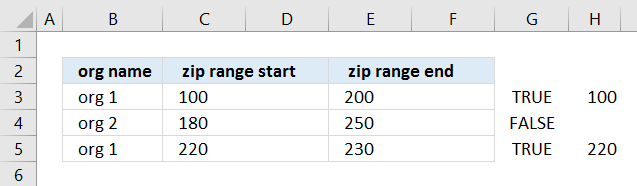#### Step 2 - Compare with list

IF(\$B15=\$B\$3:\$B\$5, \$C\$3:\$C\$5, "")<=TRANSPOSE(\$B\$8:\$B\$12)

becomes

{100; ""; 220}<=TRANSPOSE(\$B\$8:\$B\$12)

becomes

{100; ""; 220}<={100,110,190,220,225}

and returns {TRUE, TRUE, TRUE, TRUE, TRUE; FALSE, FALSE, FALSE, FALSE, FALSE; FALSE, FALSE, FALSE, TRUE, TRUE}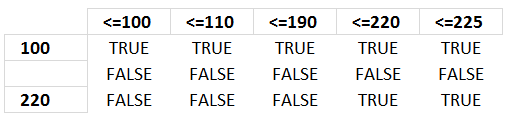#### Step 3 - Filter end range values

IF(\$B15=\$B\$3:\$B\$5, \$E\$3:\$E\$5, "")

becomes

IF("org 1 "={"org 1 ";"org 2 ";"org 1 "},{200;"";230},"")

becomes

IF({"TRUE";"FALSE";"TRUE"},{200;"";230},"")

and returns the following array: {200;"";230}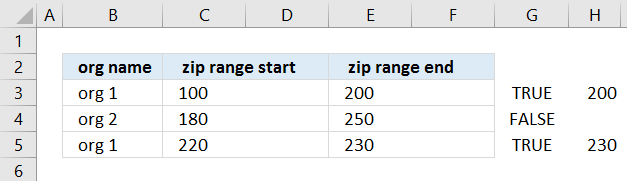#### Step 4 - Compare with list

IF(\$B15=\$B\$3:\$B\$5, \$E\$3:\$E\$5, "")>=TRANSPOSE(\$B\$8:\$B\$12)

becomes

{200;"";230}>=TRANSPOSE(\$B\$8:\$B\$12)

becomes

{200;"";230}>={100,110,190,220,225}

and returns {FALSE, FALSE, FALSE, TRUE, TRUE;FALSE, FALSE, FALSE, FALSE, FALSE;FALSE, FALSE, FALSE, FALSE, FALSE}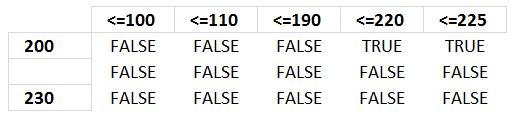#### Step 5 - Filter values

Multiplying both logical expressions gives this formula:

IF((IF(\$B15=\$B\$3:\$B\$5, \$C\$3:\$C\$5, "")<=TRANSPOSE(\$B\$8:\$B\$12))*(IF(\$B15=\$B\$3:\$B\$5, \$E\$3:\$E\$5, "")>=TRANSPOSE(\$B\$8:\$B\$12)), TRANSPOSE(\$B\$8:\$B\$12), "")

becomes

IF({TRUE, TRUE, TRUE, TRUE, TRUE; FALSE, FALSE, FALSE, FALSE, FALSE; FALSE, FALSE, FALSE, TRUE, TRUE}*{FALSE, FALSE, FALSE, TRUE, TRUE;FALSE, FALSE, FALSE, FALSE, FALSE;FALSE, FALSE, FALSE, FALSE, FALSE}, TRANSPOSE(\$B\$8:\$B\$12), "")

becomes

IF({1,1,1,0,0;0,0,0,0,0;0,0,0,1,1}, TRANSPOSE(\$B\$8:\$B\$12), "")

becomes

IF({1,1,1,0,0;0,0,0,0,0;0,0,0,1,1}, {100,110,190,220,225}, "")

and returns this array:

{100,110,190,"","";"","","","","";"","","",220,225}

The following picture shows an index table that has values horizontally and what range they are in.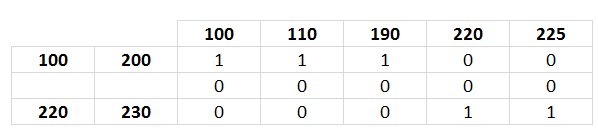Example, values in range 100-200 are 100, 110 and 190 because the array has number 1 (TRUE) in those locations.

#### Step 6 - Find the k-th smallest value

SMALL(IF((IF(\$B15=\$B\$3:\$B\$5, \$C\$3:\$C\$5, "")<=TRANSPOSE(\$B\$8:\$B\$12))*(IF(\$B15=\$B\$3:\$B\$5, \$E\$3:\$E\$5, "")>=TRANSPOSE(\$B\$8:\$B\$12)), TRANSPOSE(\$B\$8:\$B\$12), ""), COLUMNS(\$A\$1:A1))

becomes

SMALL({100,110,190,"","";"","","","","";"","","",220,225}, COLUMNS(\$A\$1:A1))

becomes

SMALL({100,110,190,"","";"","","","","";"","","",220,225}, 1)

and returns 100 in cell C15.